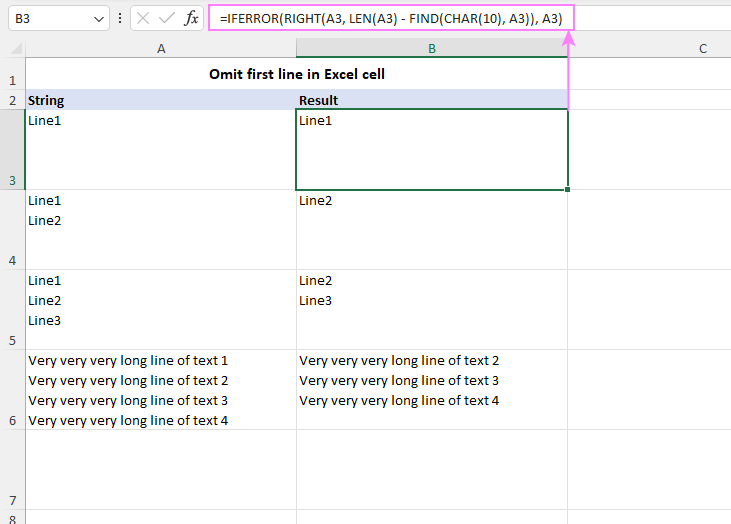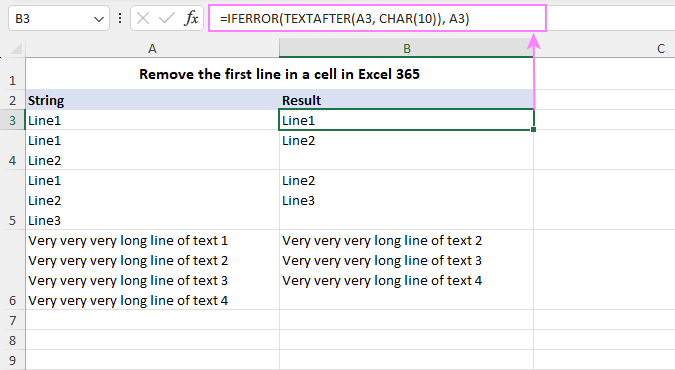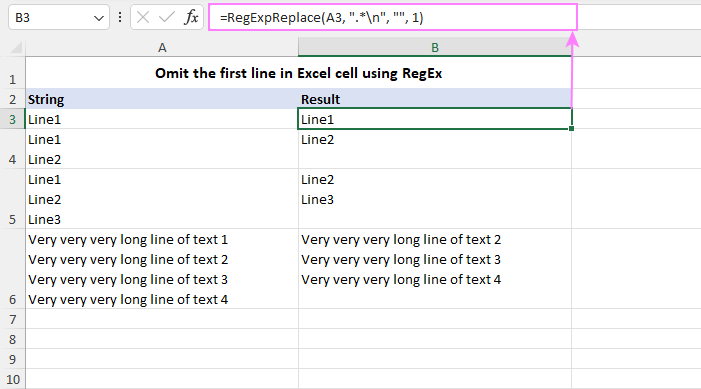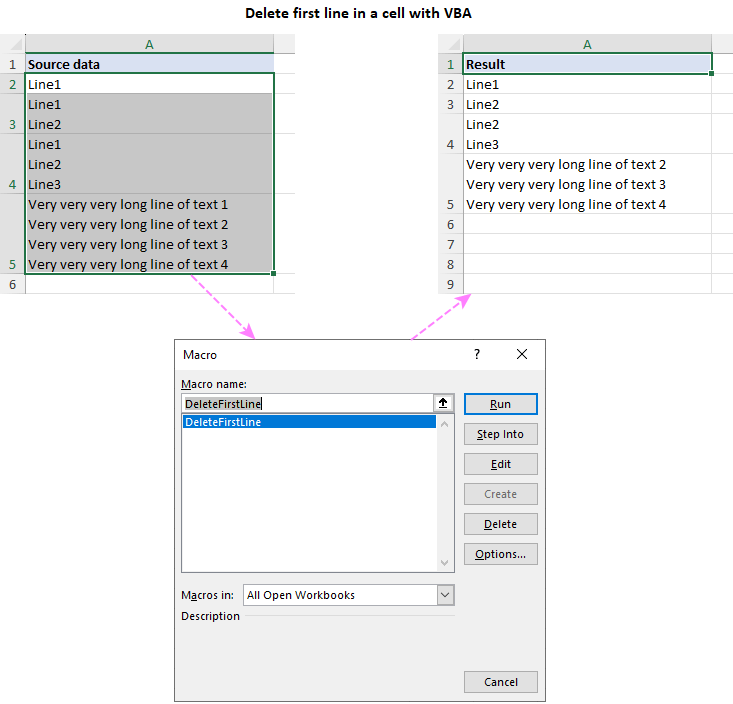# How to omit / remove the first line in Excel cell

The tutorial covers efficient methods to remove the first line in Excel cells by using native formulas, regular expressions, and VBA macro.

When dealing with multi-line data in Excel, you may come across scenarios where you need to omit the first line of text from a cell. This could be to remove headers or unnecessary information from imported data, clean up data for analysis, or simply extract relevant information. In this article, we will explore several methods to achieve this task efficiently.

## How to omit the first line in Excel cell

In situations when you need to remove the first line of text from a cell and extract the remaining lines for further analysis or presentation, a powerful formula combining four different functions can be used to achieve this task accurately:

RIGHT(cell, LEN(cell) - FIND(CHAR(10), cell))

Assuming the multi-line text you want to truncate is in cell A3, here's how you can adjust this formula to omit the first line and copy the remaining lines to an adjacent cell:

`=RIGHT(A3, LEN(A3) - FIND(CHAR(10), A3))`

### Handling one-line cells

There is a possibility that some cells in your dataset might contain only one line of text and not have a line feed character CHAR(10) in them. To prevent errors in such cells, you can wrap the formula with the IFERROR function like this:

`=IFERROR(RIGHT(A3, LEN(A3) - FIND(CHAR(10), A3)), A3)`How the formula works:

Let's break down the formula and understand how it omits the first line of text:

1. The CHAR(10) function returns the line feed character represented by number 10, which is used to identify line breaks in the text.
2. The FIND function locates the position of the line feed character within the source string, which indicates the end of the first line.
3. The LEN function calculates the total length of the original text string.
4. By subtracting the position of the line feed character from the total string length, you get the number of characters in the cell minus the first line.
5. Finally, the RIGHT function is used, where the calculated number of characters from step 4 is provided as the num_chars argument. This function returns that many characters from the right side of the text, effectively excluding the first line.

Let's illustrate the formula with an example. Suppose cell A5 contains the following 3 lines of text, each consisting of 5 characters:

Line1
Line2
Line3

The FIND(CHAR(10)) combination would return 6, which is the position of the first line feed character in the cell.

The LEN(A5) formula returns 17, which is the total number of characters in the cell (15 text chars + 2 line feed chars).

The subtraction operation 17-6 gives 11, which is the number of characters in lines 2 and 3.

The RIGHT(A5, 11) function returns 11 characters from the right side of the string in A5, which precisely omits the first line and leaves lines 2 and 3 intact.

## Remove first line of text in Excel 365

With the introduction of a variety of new functions in Excel 365, manipulating text in cells has become remarkably easy. One of the new functions, TEXTAFTER, allows you to remove the first line of text simply by specifying the original cell and the delimiter, which is obviously the line feed character:

TEXTAFTER(cell, CHAR(10))

For example, to remove the first line of text in cell A3 and extract the remaining lines into another cell, the formula is as straightforward as:

`=TEXTAFTER(A3, CHAR(10))`

In some cases, you might encounter cells with only one line of text, and using the TEXTAFTER function could lead to #N/A errors. To tackle this, just use IFERROR as a wrapper for the core formula:

`=IFERROR(TEXTAFTER(A3, CHAR(10)), A3)`

This formula ensures that if there is only one line of text in the target cell (no line feed character present), Excel will return the original text without any errors.That's all there is to it! With the TEXTAFTER function, removing the first line in an Excel cell has become a breeze :)

## Omit first line in a cell using Excel RegEx

For advanced Excel users familiar with regular expressions, there is a powerful solution available to omit the first line of text from a cell - a custom RegEx Replace function:

RegExpReplace(text, pattern, text_replace, [instance_num], [match_case])

The idea behind this function is to use a regular expression to match the text preceding the new line and replace it with an empty string:

RegExpReplace(cell, ".*\n", "", 1)

Breaking down the components of this generic formula:

• Cell- is the reference to the cell containing the multi-line text that you want to modify.
• ".*\n" - is the regular expression pattern that matches any characters (.*), followed by a line break (\n).
• "" - is the replacement text, which is an empty string, indicating that you want to remove the matched text.
• 1 - is the instance number, specifying that you look for the first occurrence of the pattern.

To be able to use this function in your Excel, its code needs to be inserted in your workbook first. You can find the code in the sample workbook at the end of this tutorial or in this article: Excel VBA Regex Replace function. Once the code is added, you can use it just like any native Excel function.

For instance, to remove the first line of text in cell A3, the formula takes this form:

`=RegExpReplace(A3, ".*\n", "", 1)`

Please pay attention that when using the RegExpReplace function, you only need to provide the correct reference in the first argument (text). The other three arguments (pattern, text_replace, instance_num) remain constant in this context.Tip. The users of our Ultimate Suite can achieve the same result effortlessly. Seamlessly integrated into Excel, the powerful Regex Tools allow you to perform all kinds of manipulations with text strings without the need for any VBA code in your workbooks.

## Delete first line in cell with Excel VBA

If you frequently need to remove the first line of text from cells in Excel, automating the process with VBA can be a time-saving solution. The following VBA code allows you to quickly delete the first line of text from the selected cell or a range of cells.

To delete the first line in a cell with VBA macro, follow these steps:

1. Add the code to your workbook as described in How to insert VBA code in Excel.
2. Select the range of cells containing the text where you want to remove the first line.
3. Press Alt + F8 to open the Macro dialog box.
4. Choose the DeleteFirstLine macro and click Run to execute the code.

Word of caution! Unlike the previous methods, this VBA code deletes the first line in the original cells. Since actions performed by Excel macros cannot be undone, it stands to reason to back up the worksheet before running the macro to ensure data integrity.Mastering different methods of omitting the first line in Excel cells opens up a world of new possibilities for efficient data management. Whether you choose to utilize formulas, leverage the power of regular expressions, or embrace VBA automation, each method empowers you to handle multi-line text with precision and ease!